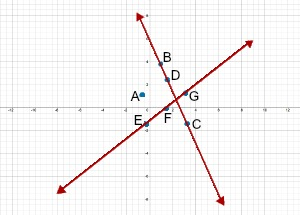# Collinear in Geometry: Definition & Example - Quiz & Worksheet

Instructions:

question 1 of 3

### Are the points (3,14), (1,8), and (8,29) collinear? If so, what is the slope of the line that they lie on.

Create Your Account To Take This Quiz

As a member, you'll also get unlimited access to over 84,000 lessons in math, English, science, history, and more. Plus, get practice tests, quizzes, and personalized coaching to help you succeed.

Try it risk-free for 30 days. Cancel anytime

### 2. Which of the following sets of points are collinear?Create your account to access this entire worksheet
Quizzes, practice exams & worksheets
Certificate of Completion
Create an account to get started

Collinear points are an important part of geometry. Check your understanding of the rules surrounding them by completing the practice problems in this quiz.

## Quiz & Worksheet Goals

You will solve problems involving:

• Determining if points are collinear
• The slope of lines formed by collinear points
• Identifying collinear points on a graph

## Skills Practiced

Strengthen the following skills:

• Reading comprehension - ensure that you draw the most important information from the related lesson on collinear in geometry
• Problem solving - use acquired knowledge to solve practice problems that involve collinear points
• Knowledge application - use your knowledge to answer questions related to finding the slope of a line through specific collinear points

Collinear points in geometry are discussed further by the lesson related to this quiz, entitled Collinear in Geometry: Definition & Example. Reading this lesson will teach you about:

• Examples of collinear points in nature and music
• Graphing collinear points
• How the slope of a line can determine collinear points
Final ExamNY Regents Exam - Integrated Algebra: Help and Review
Status: Not Started
Chapter ExamNY Regents - Graphing Functions: Help and Review
Status: Not Started

Support